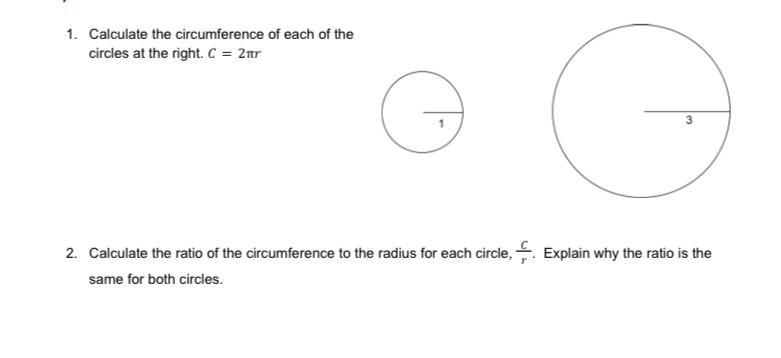Question:

# 1. Calculate the circumference of each of the circles at the right. C = 2tr 3 2. Calculate the ratio of the circumference to the1. Calculate the circumference of each of the circles at the right. C = 2tr 3 2. Calculate the ratio of the circumference to the radius for each circle, Explain why the ratio is the same for both circles.